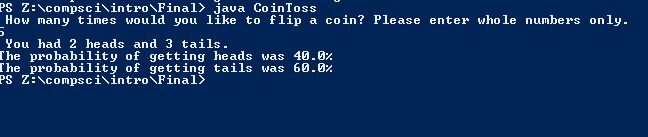# Assignment #Final

## Code

```        /// Name: Lacey Reese
/// Period: 5
/// Program Name:  Final
/// File Name:  Final.java
/// Date Finished 1/20/2016

import java.util.Scanner;

import java.util.Random;

public class CoinToss
{

public static void main(String[] args)
{
Scanner input = new Scanner(System.in);

System.out.println( "WELCOME to Lacey's Coin Toss! Please pick a number!!!! " );
int n = input.nextInt();
while ( n < 1 || n > 2100000000 )
{
System.out.println("Please select a number between 1 and 2,100,000,000.");
n = input.nextInt();
}

int f = n;
int h = 0, t = 0;

Random r = new Random();
while ( n > 0 )
{
int x = r.nextInt(2);

if ( x == 0 )
{
h++;
n--;
} else if ( x == 1 )
{
t++;
n--;
}

}
double probHeads = 100 * (double)h / f;
double probTails = 100 * (double)t / f;
System.out.println(" You had " + h + " heads and " + t + " tails.");
System.out.println( "The probability of getting heads was " + probHeads + "%" );
System.out.println( "The probability of getting tails was " + probTails + "%" );

// I used a while loop because I thought it was the easiest for me
/// I think high numbers that are  divisible by 10 can getcloser to a 50 50 ratio
}
}

Picture of the output
//```